# Classical mechanics

This is the current revision of Classical mechanics as edited by DavidB4 (Talk | contribs) at 21:20, September 8, 2020. This URL is a permanent link to this version of this page.

(diff) ← Older revision | Latest revision (diff) | Newer revision → (diff)
Jump to: navigation, search

Classical mechanics refers to a branch of physics dealing with every-day laws of mechanics (as opposed to quantum mechanics). The term is sometimes interchangeable with "Newtonian physics", as it was Sir Isaac Newton who first proposed the first laws of motion. However, there are other formulations of classical mechanics that are not due to Newton, namely Lagrangian dynamics and Hamiltonian dynamics. (These are simply different formulations of the same principles; they are precisely equivalent to Newtonian mechanics.) Classical mechanics is sufficient for explaining most observable phenomena.

## Kinematics

Kinematics is the subfield of mechanics that describes motion, without taking into account its cause. The relevant concepts in kinematics are position, velocity and acceleration. The position of an object is usually described by a vector, with respect to some reference point. That position is described as a vector means that we need several numbers to specify it. For example, we can say that object A is three meters to the right and four meters to the front from object B. Note that a single number is not enough to specify its position. We could say that object A is 5 meters from object B, but in this case we need to specify its direction, so, in any case, we need two numbers. Velocity is measures how fast position changes. As such, it is the change in position with respect to time. Velocity is also a vector. Mathematically, it is the derivative of position with respect to time:If the velocity is constant, the distance traveled in a time t is: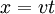.

Similarly, acceleration measures how fast the velocity changes. It is then mathematically defined as the derivative of velocity with respect to time: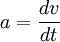If the acceleration is constant, the velocity at a time t is:.

Whereis the velocity at time t = 0. Integrating this equation gives the distance traveled by an object at constant acceleration: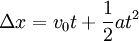.

Combining previous equations we can find a very useful relation between initial and final velocity and distance traveled, without making reference to time: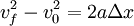## Dynamics

Dynamics is the study of the origin, or causes, of motion. The fundamental concept of dynamics is that of force. Classical dynamics is basically formulated in terms of the three Newton’s laws of motion. Newton’s laws establish the mechanical world view that forms the basis for the scientific revolution of the 17th century. Newton’s three laws are:

1. An object subject to no net external force moves with constant velocity relative to an inertial reference frame. An inertial reference frame is defined as one where Newton's laws are valid. Intuitively, an inertial reference frame is a frame that moves in a straight line at a constant velocity. Since the Earth is rotating, it is not a perfect reference frame; however, it is a very good approximation for everyday purposes.

2. In an inertial reference frame, the net force acting upon an object is equal to the rate of change of its momentum. Mathematically: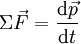whereis the net force acting on the object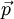is the momentum of the object

Momentum is defined as the product of mass and velocity,. If the mass of the object in question is constant, then substituting if for the momentum we find Newton's second law may be rewritten in the more familiar form of: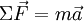where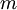is the mass of the object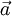is the acceleration of the object

This law also applies to a system of objects.

At first sight it seems that Newton’s first law is redundant, in the sense that it is a special case of the second law: If the force is zero, acceleration is zero, and hence the object moves at a constant velocity. However, the two laws are not redundant. The purpose of Newton’s first law is to define what an inertial reference frame is. Once defined, we can formulate Newton’s second law which is only valid on inertial reference frame.

3. For every action, there is an equal and opposite reaction.

If object A exerts a force on object B, object B will exert a force equal in magnitude and opposite in direction on object A. For example, if the earth pulls you down with a force of 1500 Newtons, you pull up on the earth with a force of 1500 Newtons. (Of course, since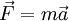, and the earth's mass is much greater than yours, the earth accelerates much less than you do.)

## Angular Kinematics

These same basic laws are true with respect to angular motion, that is, for a particle moving in a circle. In this case, the position is described by an angle, and is measured in radians. Its first derivative, ω (measured in radians/second), is called angular velocity; its second derivative α is called angular acceleration. The kinematic equations of rotational motion are analogous to those of linear motion: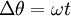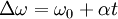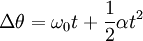## Limitations

Classical mechanics breaks down in extreme conditions, such as traveling close to the speed of light, being near extremely high gravity or dealing with very small distances as are important when dealing with subatomic particles.

### Relativity

Relativity deals with objects traveling near the speed of light or near extremely strong gravitational fields, such as black holes.

In special relativity, the Lorentz factor,, can be used to judge whether the difference between classical mechanics and relativity is significant. A value close to 1 indicates that relativistic effects are negligible.where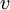is the velocity of an object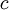is the speed of light

Speeds necessary to get a significant Lorentz factor are simply not found in real-life examples of visible objects. For example, at 5 miles per second, (18,000 miles per hour, the speed of a satellite in Earth orbit), the Lorentz factor has a value of 1.000000000360219.

### Quantum Mechanics

Quantum mechanics describes the behaviour of matter at small distances, such as those of atoms or subatomic particles. Given enough information about a classical system, we can use classical mechanics to predict how it will evolve with certainty. This is not true in quantum mechanics; in quantum mechanics, we can only calculate the probability of say the measurement of the position of a particle. This results from the wave-like nature of matter, referred to as wave-particle duality. It states that particles (such as electrons) can sometimes behave like waves and waves (such as light) can sometimes behave like particles. The reason we don't observe quantum effects on macroscopic scales can be seen by considering the equation for the wavelength,of a particle: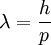whereis Planck's constant and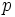is the momentum (physics) of the particle. At macroscopic scales,is very small as Planck's constant is such a small number,. Therefore, the wave-like nature of matter has little effect on macroscopic scales.

The wave-like nature of matter leads to the Heisenberg uncertainty principle, which states that there are certain quantities, such as position and momentum, that cannot be measured to an arbitrary precision simultaneously.

Particles are described by a wavefunction,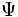, which encodes the results of all possible measurements. The wavefunction is the solution to the Schrodinger equation,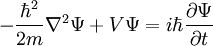where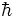is the reduced Planck's constant,is the mass of the particle and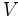is the potential the particle is in.